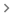Use equations in a document

You can insert mathematical equations into your documents.

Insert an equation

1. Open a document in Google Docs.
2. Click where you want to put the equation.
3. Click InsertEquation.
4. Select the symbols you want to add from one of these menus:
• Greek letters
• Miscellaneous operations
• Relations
• Math operators
• Arrows
5. Add numbers or substitute variables in the box.

To add another equation box, click New equation.

To show or hide the equation options, click ViewShow equation toolbar.

Use equation shortcuts

You can type "\" followed by the name of a symbol and a space in an equation to insert that symbol. For example, \alpha will insert 𝞪.

To type superscripts or subscripts, type "\", then press Shift + 6 or Shift + -.